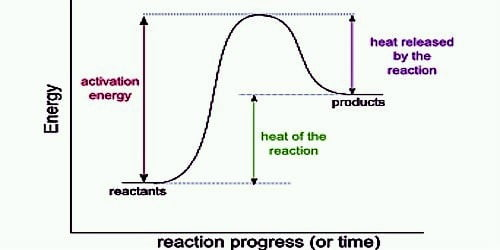# Effect of Temperature on Heat of Reaction: The Kirchhoff Equation

Effect of Temperature on Heat of Reaction: The Kirchhoff Equation

The heat change accompanying chemical or physical processes generally depends on the temperature at which the process takes place. This dependence is mathematically expressed in the form of what is known as Kirchhoff equation after G. R. Kirchhoff (1858) who first developed this equation. The equation may easily be derived with the help of the first law of thermodynamics.

Kirchhoff’s Equation is equality expressing the temperature dependence of the thermal quantities linked with a chemical reaction through the difference in heat capacities between the products and reactants. The same reaction, when carried at dissimilar temperatures, the enthalpies of reaction are also different.

Kirchhoff equation relates the heat of reaction with the definite heats of a structure before and after the reaction. Kirchhoff equation is represented as dQ/dt = C – C’

Where, Q is the heat energy evolved throughout the procedure at temperature ‘t’ without modifying in volume and C is the specific heats of the reactants and C’ is the specific heats of products.

Consider the process in which the reactants in state A at temperature T1 are converted into products in state B a temperature T2. Assume that all operations are carried out at constant pressure. The conversion may be carried out in two ways but according to Hess’s law, the total heat change must be the same in both cases.

(1) The reactants in state A at temperature T1 are heated to a temperature T2. The heat absorbed is (∆T) (CP)A. where ∆T = T2 – T1, and (CP)A is the heat capacity of the reactants in the state A. The reaction is now allowed to take place at this temperature and the heat change for the process is (HB – HA)2 = ∆H2

The total heat change for the process = (∆T) (CP)A + ∆H2.

(2) The reactants in state A at temperature T1 are considered to products in state B at the same temperature. The heat cement change = (HB – HA)1 = ∆H1. The temperature of the products is then raised from T1 to T2 and the heat absorbed is (∆T) (CP)B, where (CP)B is the heat capacity of the products.

The total heat change for the process = (∆T) (CP)B + ∆H1.

From Hess’s law,

(∆T) (CP)A + ∆H2 = (∆T) (CP)B + ∆H1

or, ∆H2 – ∆H1 = [(CP)B – (CP)A] x (∆T)

= ∆CP (∆T)

where, ∆CP = (CP)B – (CP)A

or, [∆H2 – ∆H1] / ∆T = ∆CP

For an infinitesimally small change in temperature, one can write,

[d∆H / dT] = ∆CP … … … … (1)

Similarly, it may be shown that if the process is carried out at constant volume the relationship is;

[d∆U / dT] = ∆C … … … … (2)

The relationships (1) and (2) are different forms of the Kirchhoff equation. The equations are useful for calculating the heat 41 reactions at a given temperature when the value is known at another temperature provided the heat capacities of the reactants and products are also known.

A temperature transform occurs when the temperature is increased or decreased by the flow of heat. This shifts the chemical equilibrium toward the products or reactants, which can be resolute by studying the reaction and deciding whether it is endothermic or exothermic. The equation which indicates Effect of temperature on the heat of reaction is known as Kirchhoff’s equation.